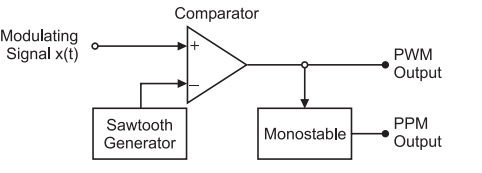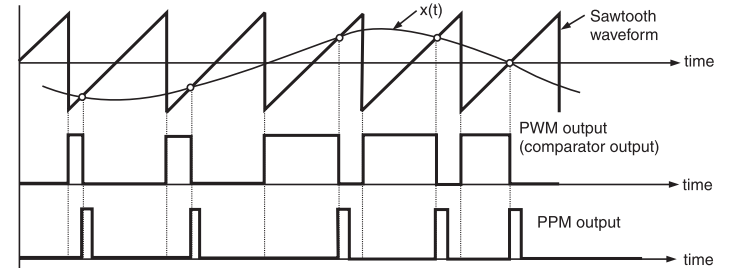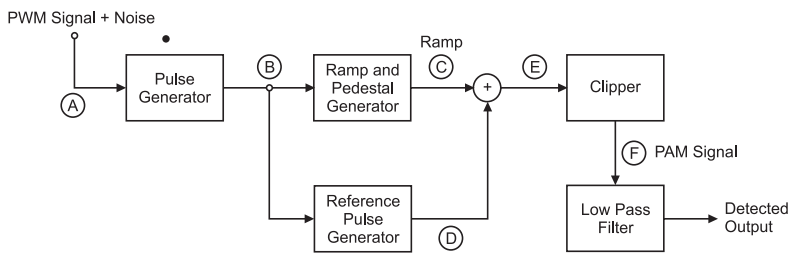# Generation and Detection of a PWM Signal

## Pulse Width Modulation (PWM)

In PWM, the width of the modulated pulses varies in proportion with the amplitude of modulating signal. The waveforms of PWM is shown in fig.1 below.Fig.1 : PWM signal

As we can observe, the amplitude and the frequency of the PWM wave remain constant. Only the width changes.

That is why the information is contained in the width variation. This is similar to FM.

As the noise is normally additive noise, it changes the amplitude of the PWM signal.

At the receiver, it is possible to remove these unwanted amplitude variations very easily by means of a limiter circuits.

As the information is contained in the width variation, it is unaffected by the amplitude variations introduced by the noise. Thus, the PWM system is more immune to noise than the PAM signal.

### Generation of PWM Signal

The block diagram of a PWM signal generator is shown in fig.2 below. This circuit can also be used for the generation of PPM signal.Fig.1 : PWM and PPM Generator

• A sawtooth generator generates a sawtooth signal of frequency fs, and this sawtooth signal in this case is used as a sampling signal.
• It is applied to the inverting terminal of a comparator.
• The modulating signal x (t) is applied to the non-inverting terminal of the same comparator.
• The comparator output will remain high as long as the instantaneous amplitude of x (t) is higher than that of the ramp signal.
• This gives rise to a PWM signal at the comparator output as shown in fig.2 .Fig.2 : Waveforms

Here, it may be noted that the leading edges of the PWM waveform coincide with the falling edges of the ramp signal. Thus, the leading edges of PWM signal are always generated at fixed time instants.

However, the occurance of its trailing edges will be dependent on the instantaneous amplitude of x(t). Therefore, this PWM signal is said to be trail edge modulated PWM.

### Detection of PWM Signal

The circuit for the detection of PWM signal is shown in fig.3 below.Fig.3 : PWM Detection Circuit

The working operation of the circuit may be explained as under:

• The PWM signal received at the input of the detection circuit is contaminated with noise. This signal is applied to pulse generator circuit which regenerates the PWM signal.
• Thus, some of the noise is removed and the pulses are squared up.
• The regenerated pulses are applied to a reference pulse generator. It produces a train of constant amplitude, constant width pulses.
• These pulses are synchronized to the leading edges of the regenerated PWM pulses but delayed by a fixed interval.
• The regenerated PWM pulses are also applied to a ramp generator. At the output of it, we get a constant slope ramp for the duration of the pulse. The height of the ramp is thus proportional to the width of the PWM pulses.
• At the end of the pulse, a sample and hold amplifier retains the final ramp voltage until it is reset at the end of the pulse.
• The constant amplitude pulses at the output of reference pulse gtenerator are then added to the ramp signal.
• The output of the adder is then clipped off at a thereshold level to generate a PAM signal at the output of the clipper.
• A low pass filter is used to recover the original modulating signal back from the PAM signal. The waveforms for this circuit have been shown in fig.4.Fig.4 : Waveforms for PWM detection circuit